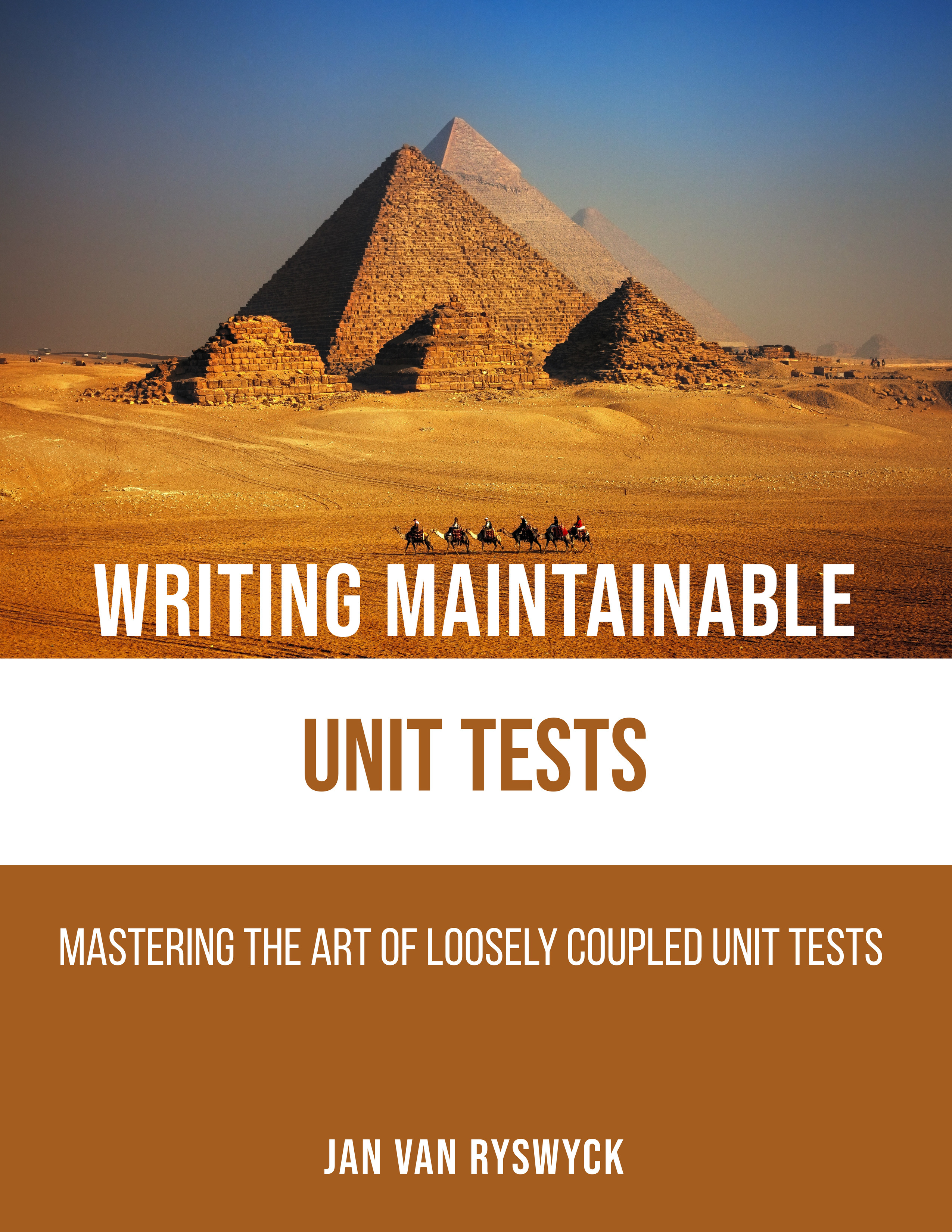# Blog

## Clojure Kata #3 – Roman Numerals

April 29, 2014

I’m still in the process of trying to wrap my head around Clojure. I’ve been practicing several different katas like Fizz Buzz and the Bowling Game. Another one I’ve been doing a lot lately is the Roman Numerals kata. This exercise combines both my fascination for Ancient Rome and learning a programming language.

Here is the code of my latest stab at this problem:

``````(ns roman_numbers.core
(:require [clojure.math.numeric-tower :as math])
(:require [clojure.string :as str]))

(def symbols (sorted-map-by > 1000 "M" 500 "D" 100 "C" 50 "L" 10 "X" 5 "V", 1 "I"))

(defn orderOfMagnitude [number]
(math/expt 10 (count (str number))))

(defn determineApplicableSymbols [number]
(let [magnitude (orderOfMagnitude number)]
(if(<= magnitude 1000)
(take 3 (filter (fn[x] (>= magnitude (first x)))
symbols))
{ -1 "-" -2 "-" 1000 "M" } )
))

(defn selectSymbol [number unitSymbol fiveSymbol tenSymbol]
(let [unitSymbolValue (key unitSymbol)
unitNumber (quot number unitSymbolValue)
total (* unitNumber unitSymbolValue)]

(cond
(< unitNumber 4)
(first {
total
(apply str
(repeat unitNumber (val unitSymbol)))
})

(< unitNumber 9)
(first { total
(str/join
(concat
(repeat (- 5 unitNumber)
(val unitSymbol))
(val fiveSymbol)
(repeat (- unitNumber 5)
(val unitSymbol)))
)})

(= unitNumber 9)
(first { total (apply str (val unitSymbol)
(val tenSymbol)) })
)
))

(defn convert [number]
(loop [currentNumber number
result ""]
(if (zero? currentNumber)
result
(let [applicableSymbols (determineApplicableSymbols
currentNumber)
unitSymbol (last applicableSymbols)
fiveSymbol (second applicableSymbols)
tenSymbol (first applicableSymbols)
matchingSymbol (selectSymbol
currentNumber unitSymbol
fiveSymbol tenSymbol)]
(recur (- currentNumber (key matchingSymbol))
(str result (val matchingSymbol)))
))))
``````

This might seem a bit overwhelming, but don’t let that scare you. Let me explain the gist of this piece of code. When the convert function is called with a particular number, let’s say 14, first thing the applicable symbols are determined by calling the function determineApplicableSymbols. These are taken from the symbols map depending on the size of the specified number. For this first iteration, the applicable symbols are returned in a sequence containing [100 C] [50 L] [10 X].

The actual meat of the conversion functionality is contained by the selectSymbol function. Calling this function returns a matching symbol, which in this case returns [10 X]. The number 10 is subtracted from our initial number and the symbol X is added to the result. Then the recursion starts again, but now currentNumber is assigned to the number 4 and result contains the string “X”.

For the second iteration, the applicable symbols are [10 X] [5 V] [1 I] where the selectSymbol function converts the number 4 into “IV” which is added to the result.

In the third and final iteration, the currentNumber is 0 which breaks out of the loop and returns the result, which is “XIV”.

For completeness sake, here are the unit tests:

``````(ns roman_numbers.t-core
(:use midje.sweet)
(:use [roman_numbers.core]))

(facts "When converting an Arabic number to a Roman Number"
(fact "it returns I for the number 1"
(convert 1) => "I")

(fact "it returns II for the number 2"
(convert 2) => "II")

(fact "it returns III for the number 3"
(convert 3) => "III")

(fact "it returns IV for the number 4"
(convert 4) => "IV")

(fact "it returns V for the number 5"
(convert 5) => "V")

(fact "it returns VIII for the number 8"
(convert 8) => "VIII")

(fact "it returns IX for the number 9"
(convert 9) => "IX")

(fact "it returns X for the number 10"
(convert 10) => "X")

(fact "it returns XIV for the number 14"
(convert 14) => "XIV")

(fact "it returns XXXIX for the number 39"
(convert 39) => "XXXIX")

(fact "it returns XLIX for the number 49"
(convert 49) => "XLIX")

(fact "it returns CCLXXVIII for the number 278"
(convert 278) => "CCLXXVIII")

(fact "it returns CML for the number 950"
(convert 950) => "CML")

(fact "it returns MDCCCXLV for the number 1845"
(convert 1845) => "MDCCCXLV")

(fact "it returns MMCCCLXXVIII for the number 2378"
(convert 2378) => "MMCCCLXXVIII")
)
``````

There are probably better and more idiomatic solutions to solve this problem in Clojure or other programming languages. I would love to see some other solutions as well.

Until next time.

If you and your team want to learn more about how to write maintainable unit tests and get the most out of TDD practices, make sure to have look at our trainings and workshops or check out the books section. Feel free to reach out at infonull@nullprincipal-itnull.be.#### Jan Van Ryswyck

Thank you for visiting my blog. I’m a professional software developer since Y2K. A blogger since Y2K+5. Provider of training and coaching in XP practices. Curator of the Awesome Talks list. Past organizer of the European Virtual ALT.NET meetings. Thinking and learning about all kinds of technologies since forever.### Latest articles

• ###### Contract Tests - Parameterised Test Cases

June 28, 2023

• ###### Contract Tests - Abstract Test Cases

April 12, 2023

• ###### Contract Tests

February 1, 2023

June 15, 2022

• ###### Tales Of TDD: The Big Refactoring

February 2, 2022

### Disclaimer

The opinions expressed on this blog are my own personal opinions. These do NOT represent anyone else’s view on the world in any way whatsoever.

Thank you for visiting my website. I’m a professional software developer since Y2K. A blogger since Y2K+5. Author of Writing Maintainable Unit Tests. Provider of training and coaching in XP practices. Curator of the Awesome Talks list. Thinking and learning about all kinds of technologies since forever.

June 28, 2023

April 12, 2023

February 1, 2023